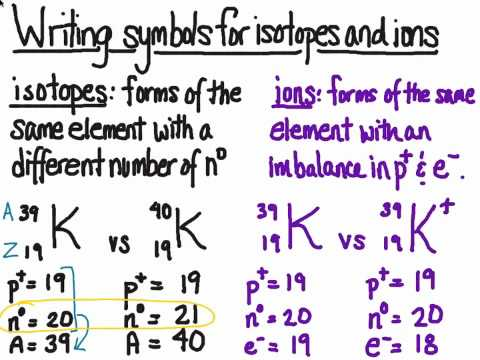# Isotopes

## Key Questions

• Every isotope (at least, the ones that occur naturally) contributes to the average atomic mass, which appears in the element's box on most periodic tables. But the average is what is called a weighted average.

A weighted average mass is an average that takes into account how many times each mass occurs in a sample. For example, if 10 people in your class have a mass of 50 kg and 20 people have a mass of 60 kg, what will be the average mass?

You might be tempted to add 50 and 60 and divide by 2 for an average of 55 kg. THAT WOULD BE INCORRECT. You must find the total mass (500 + 1200 = 1700) and divide it by the total number of people (30) so the average is 56.67. Notice the average is closer to the mass that had more people.

You could do the same thing using percentages. 33.33% of the class has a mass of 50 kg and 66.67% has a mass of 60 kg. Use decimals for the percentages:

$$ .3333 x 50 = 16.665
.6667 x 60 = 40.02


If we add these two masses we arrive at 56.68. The answer is a little different because of the rounding.

Both processes use the same steps (adding and multiplying) but in a different order.

The second process is the one normally used in determining average atomic mass. It is possible to measure what percentage of an element's sample is made of each isotope. Then the weighted average is calculated and printed on a periodic table.

EXAMPLE

Silver consists of 51.86 % Ag-107 (atomic mass 106.9 u) and 48.14 % Ag-109 (atomic mass 108.9 u). What is its average atomic mass?

Solution

0.5184 × 106.9 u = 55.42 u
0.4816 × 108.9 u = 52.45 u
Weighted avge. = 107.87 u

The average atomic masses on a periodic table are usually not a nice whole number. In elements with very high atomic numbers you will see whole number atomic masses. These are not average masses; they are the mass of the most stable isotope.

(Another reason that the average atomic masses are not whole numbers is because the isotopes' masses are not whole numbers. That has to do with something called binding energy and also with the fact the the masses of protons and neutrons are not exactly 1 atomic mass unit. But I think that is beyond the scope of this question.)

Here is a video discussion of how to do this type of calculation.

video from: Noel Pauller

• Isotopes differ in the number of neutrons; in ions the number of electrons is different from the number of protons.

Isotopes are atoms that have the same number of protons but different numbers of neutrons.

Thus, atoms of $\text{_6^12"C}$ and of $\text{_6^13"C}$ are isotopes of each other. They both contain 6 protons, but one contains 6 neutrons and the other contains 7 neutrons.

Ions are atoms that have either gained or lost electrons.

Every atom has the same number of electrons and protons.

If an atom gains an electron, it gets a negative charge. If it loses an electron, it gets a positive charge. All ions have a charge.

If a carbon atom gains an electron, it becomes a C⁻ ion. If it loses an electron, it becomes a C⁺ ion.• An isotope is a variation of an element where the atom contains the same number of protons and electrons, but differs in the number of neutrons it contains in the nucleus.For example this shows three isotopes of Hydrogen

video from: enter link description here